# Equipment

## Math Lab Equipments for School

This Math Lab Equipments are going to make math easy and more results-oriented and students are going to love these types of equipment up to the level of understanding math easily. The Math Lab developed by IITians will bring revolution to the field of Math. The Math Lab is developed as per the syllabus of NCERT from classes 6th to 10th.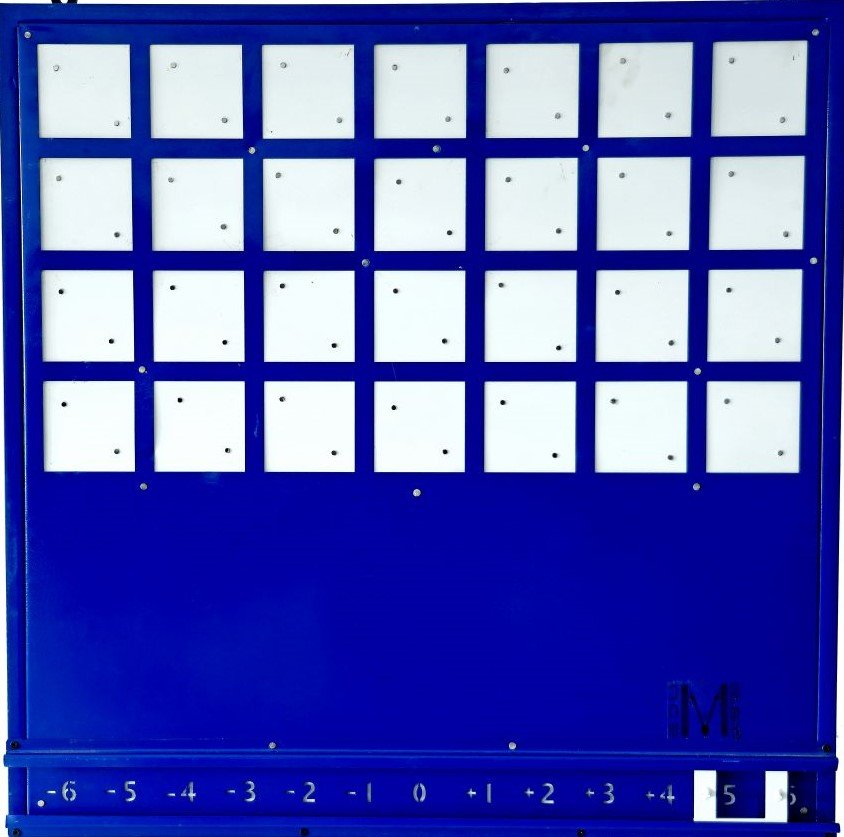# MA-01: ALGEBRA ANNOTATOR

The biggest challenge in learning Algebra is understanding the operations such as addition, subtraction, multiplication, and division of the variables and functions of the variables. The Algebra annotator is designed for learning by visualizing and doing activities involving variables. This is one of the finest math lab equipment and model for Algebra.

Chapters, Algebra

Chapters, Simple Equation, Algebraic expressions, Exponents and Powers

Chapters, Linear equation in one variable, Algebraic Expressions, Exponents and Powers, Factorisation

Chapters, Polynomial, Probability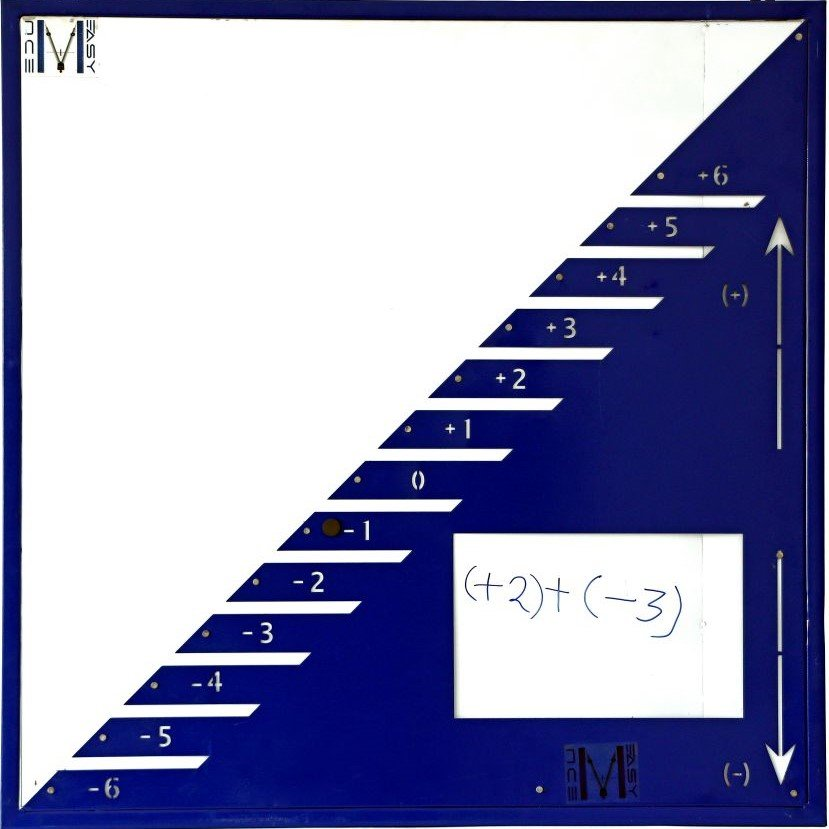# MA-02: NUMBER SYSTEM

A positive or a negative outcome is always a problem in using operations between Numbers. This operator helps in learning the concept by doing the experiments. This equipment helps in understanding the ascending & descending orders & various closer property

Chapters, Whole Numbers, Integers

Chapters, Integers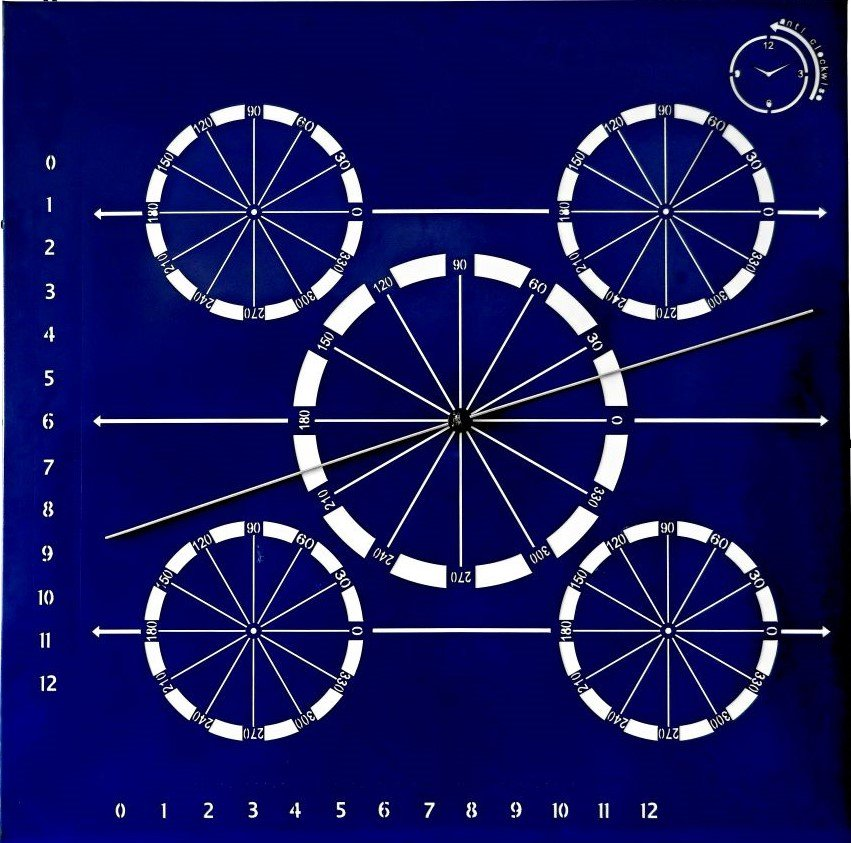# MA-03: GEOMETRY EXPOSITOR

Bothered about understating the statements and proofs of theorems! No worries, this one piece of equipment is the solution bank of all Geometrical concepts. Starting from understating the angles between two lines to visualizing the properties of parallel lines and triangles, this single equipment is loaded with a number of activities to excite the students. This equipment is it even serves the purpose of learning trigonometry.# MA-04: CO-ORDINATE SYSTEM DEMONSTRATOR

The basis of Co-ordinate Geometry is the coordinate system. For understanding the different quadrants and coordinates in a two-dimensional plane, this system demonstrates several different properties to visualize Co-ordinate Geometry. It helps in understanding the solutions of equations, roots of polynomials etc.

Chapters, Prime and composite number, Decimals, Ratio and Proportion

Chapters, Rational Numbers

Chapters, Rational numbers, Square and square roots, Cube and cube roots, Direct and Inverse proportion

Chapters, Number system, Co-ordinate geometry, Linear Equation in two variables

Chapters, Real numbers, Polynomials, Pair of equations, Quadratic equation, Arithmetic Progression, Co-ordinate geometry, Statistics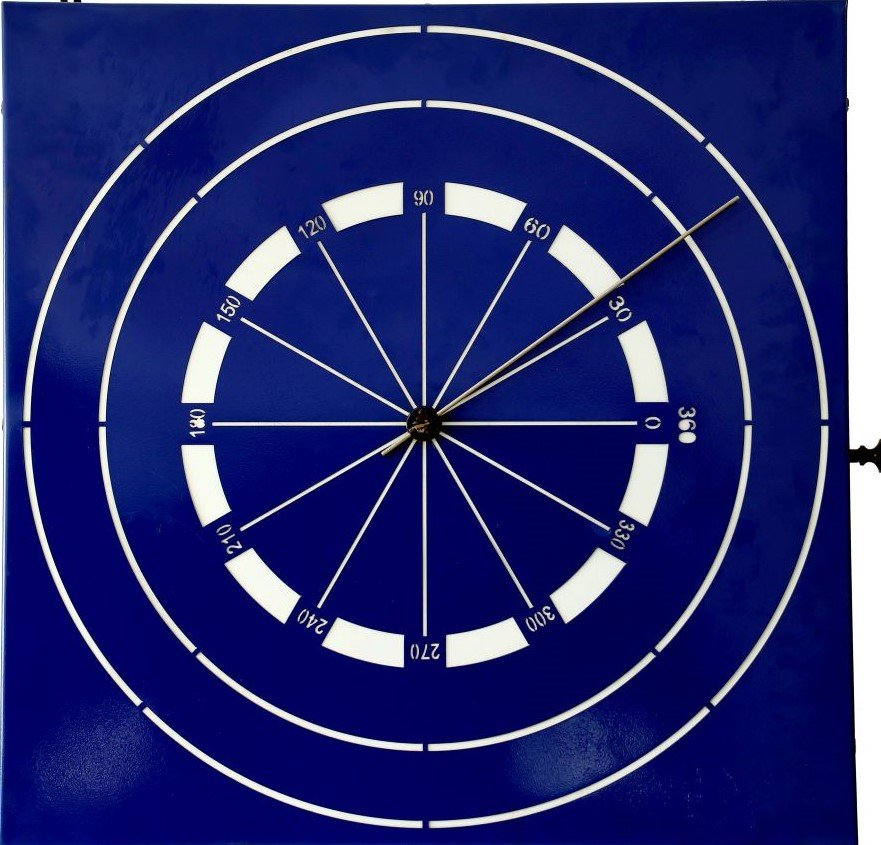# MA-05: CIRCLE DESCRIPTOR

It starts and ends at the same point. The circle is one of the most beautiful geometrical figures which has several good characteristics. However, understating the properties and theorems is a herculean task. The descriptor is designed for doing some experiments for learning the Circle in a linear manner. The descriptor is also useful in learning fractions & elementary probability.

Chapters, Comparing quantities

Chapters, Comparing quantities

Chapters, Circles

Chapters, Circles, Area Related to Circle, Probability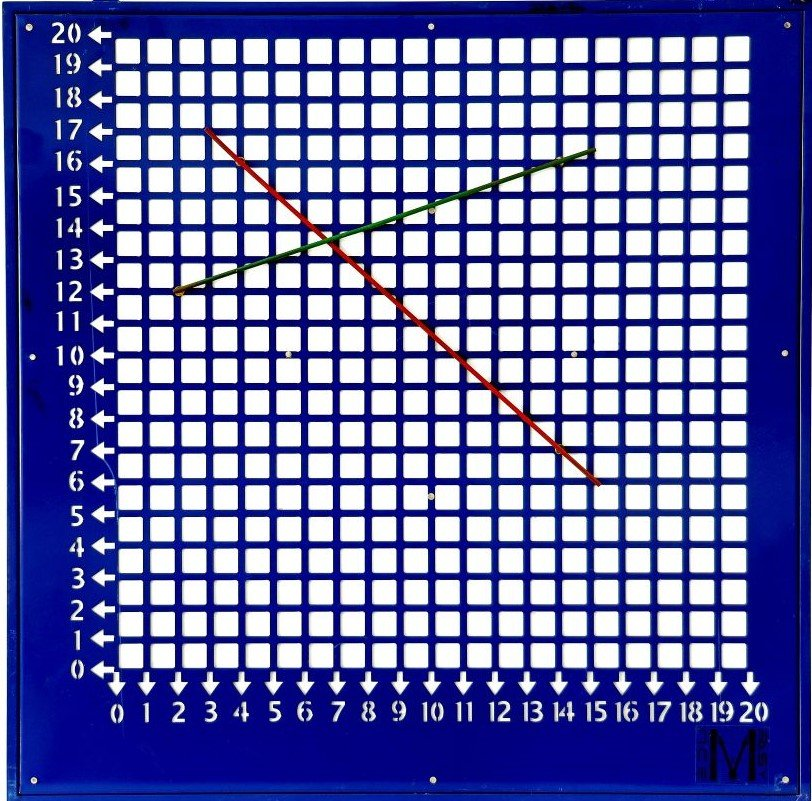# MA-06: MENSURATION EVALUATOR

Measurement of the area & volume of solids is one of the most interesting exercises in Mathematics if understood properly. The evaluator will help the teacher to demonstrate the concepts and will also help the students to learn the concepts by doing some hands-on. This math lab equipment is named as mensuration evaluator.

Chapters, Playing with numbers, Data Handling

Chapters, Data handling, Perimeter and area,

Chapters, Introduction to graphs, Mensuration

Chapters, Herons formula, Statistics, Area of a parallelogram and triangle

## Manuals of Math Lab

“Complete Guide for Teachers about Math Lab, Chapter wise as per syllabus“